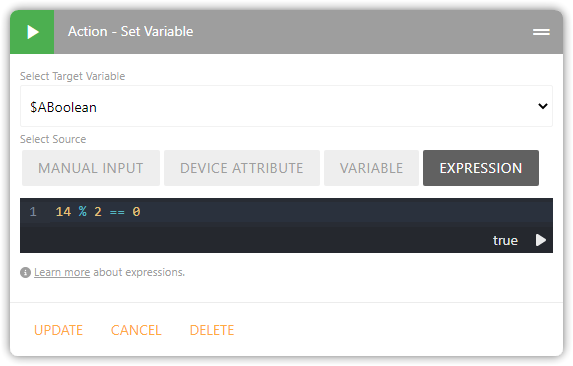# Comparison and analysis parameters for a variable

Hello to all. One tool that might be useful is to insert between the parameters of comparison for a variable, even the analysis if the (numerical) value is even and odd.

Hi Luke-
Welcome to the community and thanks for sharing your feedback! Can you give an example of what you’re trying to accomplish? I suspect it may be possible with Expressions.

For example, if you wanted to determine if something was even or odd, you could do it with a modulo math expression.

``````\$myNumber % 2 == 0
``````

In the example above, as long as `\$myNumber` is exactly divisible by two, we know it must be an even number (eg. the remainder after dividing by 2 is exactly 0).Or are you suggesting that you want the ability to run an expression directly in an IF Condition rather than first having to save it to a variable?

Hello and thank you for the answer. I am trying to make a dashboardfor the collection of waste sorted that I notice any waste I have to take out.I had thought to create a number of variables (one for type of waste)to increase every time in my neighborhood there is the collection.This because? Some types of waste have every 15 days and another 2 times a week. Increasing the variable at each waste output, I analyzed it and if it is an even number then it is up to that waste if it is an odd number I do not have to go out for collection. I don’t know if I’m making myself understood. I’m not very familiar with the English language. Sorry about the mistakes or misunderstandings.

In that case, would the example with Expressions shown above help?

Yes very useful thanks. But I haven’t found how to assign the temperature value of a device to a variable. It is feasible?

In my original screenshot above, it’s the ‘Device Attribute’ option. So you might do it in two steps:

Or if the temperature is the only trigger for the rule, you can use the Context Variable for the Event Value: `\$context.event.value`

1 Like

Thank you so much really was what I was looking for. Congratulations!